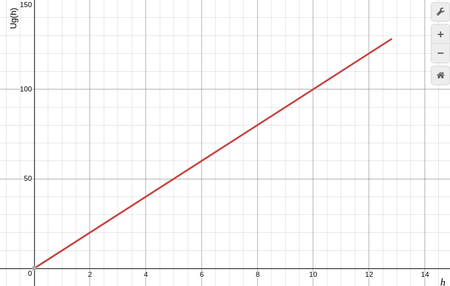# Draw a line on the graph representing the total energy E of the ball

A 1.00 kg ball is thrown directly upward with an initial speed of 16.0 m/s. A graph of the ball’s gravitational potential energy vs. height Ug(h), for an arbitrary initial velocity, is given in Part A. The zero point of gravitational potential energy is located at the height at which the ball leaves the thrower’s hand. For this problem, take g=10.0m/ s2 as the acceleration due to gravity.

Part A Draw a line on the graph representing the total energy E of the ball.
Graph of Potential Energy vs. HeightPart B Using the graph, determine the maximum height reached by the ball.